Like   Tweet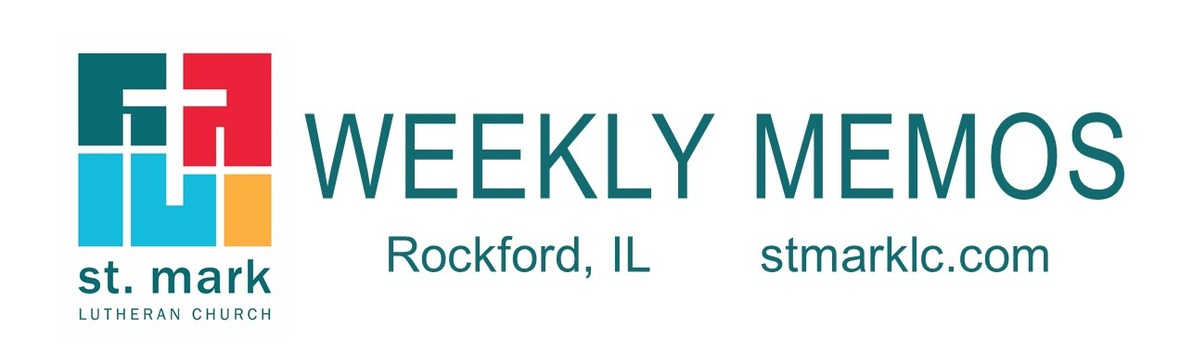/* styles */
 table div table+table+table div table{width:100%;padding:0}table div table+table+table div table img{width:96.23%;padding:0;float:none}table div table+table+table div table td{width:100%;padding:0 1.88% 18px}/* styles */## Sunday, October 6

8:00 A.M. Worship
9:30 A.M. Worship
9:30 A.M. Baptism of Clara Bruksch
9:30 A.M. Sunday School
10:45 A.M. New Member Orientation
11:00 A.M. Worship
2:00 P.M. Northern Illinois Synod - Fall Gathering at St. Mark

## Monday, October 7

9:00 A.M. Classic Chorale Rehearses
4:00 P.M. Deadline for MEMOS articles to be submitted
4:30 P.M. Yoga - in the cafe
6:30 P.M. Blue Lights
6:30 P.M. Emotions Anonymous

## Tuesday, October 8

10:00 A.M. Staff Meeting
11:00 A.M. St. Mark Duplicate Bridge
6:30 P.M. Worship & Music Ministry

## Wednesday, October 9

9:00 A.M. Women's Bible Study
9:00 A.M. Men's Bible Study
9:00 A.M. Small Bell Group
9:30 A.M. St. Mark Ringers
10:15 A.M. Altar Guild
12:30 P.M. Miriam Circle
4:30 P.M. Outreach Ministry
5:30 P.M. Good News Bearers
6:00 P.M. Bible Study
6:30 P.M. Confirmation
7:00 P.M. Voices of St. Mark

## Thursday, October 10

7:30 A.M. Panera Book Study
9:00 A.M. Cherry Valley Bridge Group
9:30 A.M. Ruth Circle
1:00 P.M. Sew and Sews
6:00 P.M. Diakonia
6:30 P.M. Emotions Anonymous
7:00 P.M. Praise Band Rehearses

## Friday, October 11

7:30 A.M. St. Mark Men's Breakfast at Denny's

## Sunday, October 13

8:00 A.M. Worship
9:30 A.M. Worship
9:30 A.M. Sunday School
10:45 A.M. New Members Orientation
11:00 A.M. Worship
7:00 P.M. 2nd Sunday at Katie's Cup

 table div table+table+table+table+table+table+table div table{width:100%;padding:0}table div table+table+table+table+table+table+table div table img{width:96.23%;padding:0;float:none}table div table+table+table+table+table+table+table div table td{width:100%;padding:0 1.88% 18px}/* styles */## News and Events

 table div table+table+table+table+table+table+table+table+table div table{width:100%;padding:0}table div table+table+table+table+table+table+table+table+table div table img{width:96.23%;padding:0;float:none}table div table+table+table+table+table+table+table+table+table div table td{width:100%;padding:0 1.88% 18px}/* styles */Hiring of New Property Manager
I am pleased to announce the hiring of Chris Semmelroth as our new Property Manager, effective Friday, October 4. Chris is a long-time member of St. Mark and has played guitar at our 11:00 service for many years. I'm quite impressed with Chris' experience, skillset, and attitude for this important position. Please join me in welcoming Chris to our staff! ~Walking this journey of faith with you, Pastor Mark

 table div table+table+table+table+table+table+table+table+table+table+table div table{width:100%;padding:0}table div table+table+table+table+table+table+table+table+table+table+table div table img{width:96.23%;padding:0;float:none}table div table+table+table+table+table+table+table+table+table+table+table div table td{width:100%;padding:0 1.88% 18px}/* styles */Healthy Family Forum
Healthy Family Forum is Wednesday, October 23, at 6:30-7:30 P.M. Parents and youth are invited to this informational learning and sharing time. We will have a panel of professional parents from the health care field that will be answering questions, sharing information and even sharing some helpful time-saving ideas for families. Questions? Need to know more before you attend? Please e-mail melinda@stmarklc.com or call 815-871-0390.

 table div table+table+table+table+table+table+table+table+table+table+table+table+table div table{width:100%;padding:0}table div table+table+table+table+table+table+table+table+table+table+table+table+table div table img{width:96.23%;padding:0;float:none}table div table+table+table+table+table+table+table+table+table+table+table+table+table div table td{width:100%;padding:0 1.88% 18px}/* styles */Are you set up for automatic giving?
In order to make giving easier than ever, we are moving to a new online giving platform. With Pushpay, you will be able to support the ministries of St. Mark securely and efficiently. For those of you that have automatic giving set up already, you should have received an email regarding the change. If you have any questions or need assistance in processing your change, please contact Wendy Iversen in the office at 815-398-3557 or finance@stmarklc.com she is more than happy to do the change for you.

During this transition, all recurring gifts with our old platform need to be cancelled. After you have cancelled your old gift, don't forget to set up a new one through the red Give button. Thank you so much for your understanding and support of St. Mark.

You can also give any time with your phone by texting STMARKLC GIVE to 77977.

 table div table+table+table+table+table+table+table+table+table+table+table+table+table+table+table div table{width:100%;padding:0}table div table+table+table+table+table+table+table+table+table+table+table+table+table+table+table div table img{width:96.23%;padding:0;float:none}table div table+table+table+table+table+table+table+table+table+table+table+table+table+table+table div table td{width:100%;padding:0 1.88% 18px}/* styles */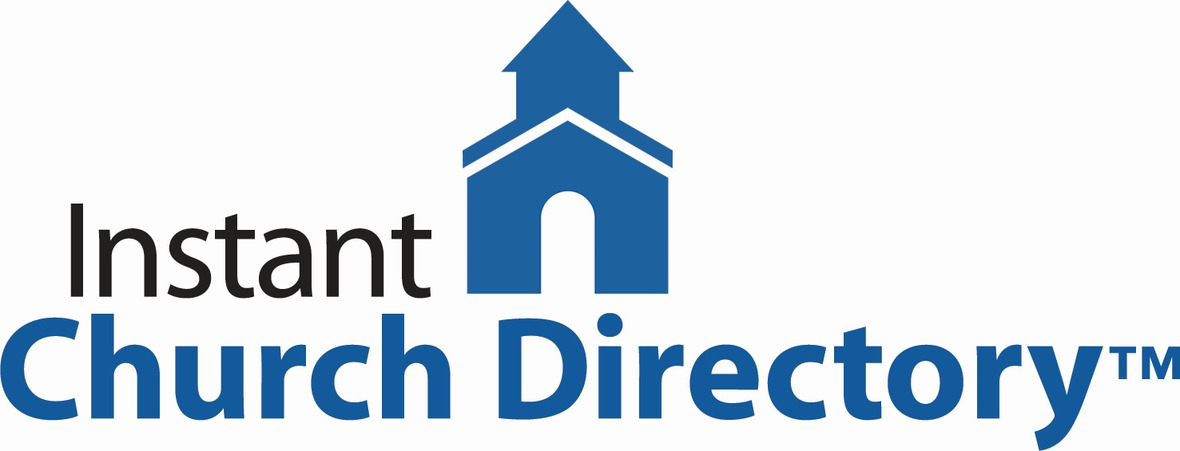Our online church directory is looking better and better every day. If you have not submitted your family photo please do so by following the link below. Also, you can download the Instant Church Directory app now.

Instant Church Directory

Once you have downloaded the app and created an account, you are not able to change or update your information, the system administrator is the only one to change and update the information. If something is incorrect, please contact the church office at 815-398-3557 or email jill@stmarklc.com.

Instant Church Directory is a simple way to view your Church Directory. Never deal with an outdated directory again, any changes made by church staff are immediately synced to the app.

Mobile App Login Instructions for Android & Kindle Devices

We also have a form for you to complete to make sure we have the most current information for the directory. There are paper forms at church or click on the link to Church Directory Form

 table div table+table+table+table+table+table+table+table+table+table+table+table+table+table+table+table+table div table{width:100%;padding:0}table div table+table+table+table+table+table+table+table+table+table+table+table+table+table+table+table+table div table img{width:96.23%;padding:0;float:none}table div table+table+table+table+table+table+table+table+table+table+table+table+table+table+table+table+table div table td{width:100%;padding:0 1.88% 18px}/* styles */Some of you have expressed concern about not having access to the new online church directory and wondering if we could print one for you. The answer is yes. We will be printing a few directories for those who have no access online or have an email account. We will let you know when this will be.

 table div table+table+table+table+table+table+table+table+table+table+table+table+table+table+table+table+table+table+table div table{width:100%;padding:0}table div table+table+table+table+table+table+table+table+table+table+table+table+table+table+table+table+table+table+table div table img{width:96.23%;padding:0;float:none}table div table+table+table+table+table+table+table+table+table+table+table+table+table+table+table+table+table+table+table div table td{width:100%;padding:0 1.88% 18px}/* styles */Calling All Musicians!
Bob Rub would like to put together an instrumental ensemble to play and accompany some pieces at St. Mark’s Christmas concert on December 8, 2019. The concert is at 3:00 p.m. If you play an instrument and would like to be a part of the concert, please email Bob at bob@stmarklc.com. In general, High school-aged youth through adults are encouraged to participate. Younger students who play and read music well will also be welcome. There will be a few rehearsals, but we can work out scheduling when we know who is available. Thank you for your consideration.

 table div table+table+table+table+table+table+table+table+table+table+table+table+table+table+table+table+table+table+table+table+table div table{width:100%;padding:0}table div table+table+table+table+table+table+table+table+table+table+table+table+table+table+table+table+table+table+table+table+table div table img{width:96.23%;padding:0;float:none}table div table+table+table+table+table+table+table+table+table+table+table+table+table+table+table+table+table+table+table+table+table div table td{width:100%;padding:0 1.88% 18px}/* styles */Panera Book Study Beginning October 10
Join Pastor Mark as he begins Panera Book Study on October 10. They meet at Panera at 609 S. Perryville, Rockford at 7:30 A.M. on Thursdays. The book will be Incarnation by William H. Willimon.

 table div table+table+table+table+table+table+table+table+table+table+table+table+table+table+table+table+table+table+table+table+table+table+table div table{width:100%;padding:0}table div table+table+table+table+table+table+table+table+table+table+table+table+table+table+table+table+table+table+table+table+table+table+table div table img{width:96.23%;padding:0;float:none}table div table+table+table+table+table+table+table+table+table+table+table+table+table+table+table+table+table+table+table+table+table+table+table div table td{width:100%;padding:0 1.88% 18px}/* styles */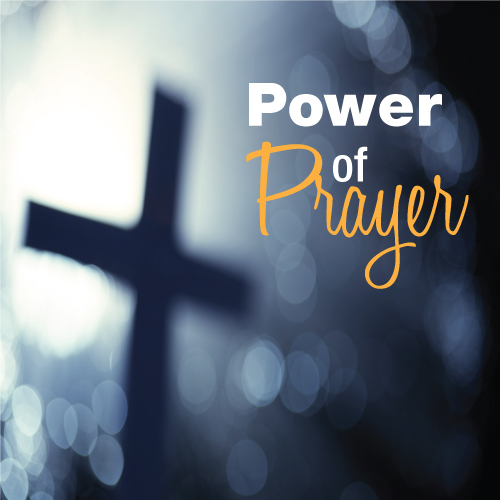St. Mark Prayer Chain
Would you like to be a part of the St. Mark prayer chain? Prayer requests come into the church office weekly. As we receive prayer requests we would send out an e-mail and ask that you pray daily for the persons mentioned in the requests. All requests are to remain confidential. Please e-mail melinda@stmarklc.com if you would like to participate in the prayer chain. “Be anxious for nothing, but in everything by prayer and supplication, with thanksgiving, let your requests be made known to God.” Colossians 4:2.

 table div table+table+table+table+table+table+table+table+table+table+table+table+table+table+table+table+table+table+table+table+table+table+table+table+table div table{width:100%;padding:0}table div table+table+table+table+table+table+table+table+table+table+table+table+table+table+table+table+table+table+table+table+table+table+table+table+table div table img{width:96.23%;padding:0;float:none}table div table+table+table+table+table+table+table+table+table+table+table+table+table+table+table+table+table+table+table+table+table+table+table+table+table div table td{width:100%;padding:0 1.88% 18px}/* styles */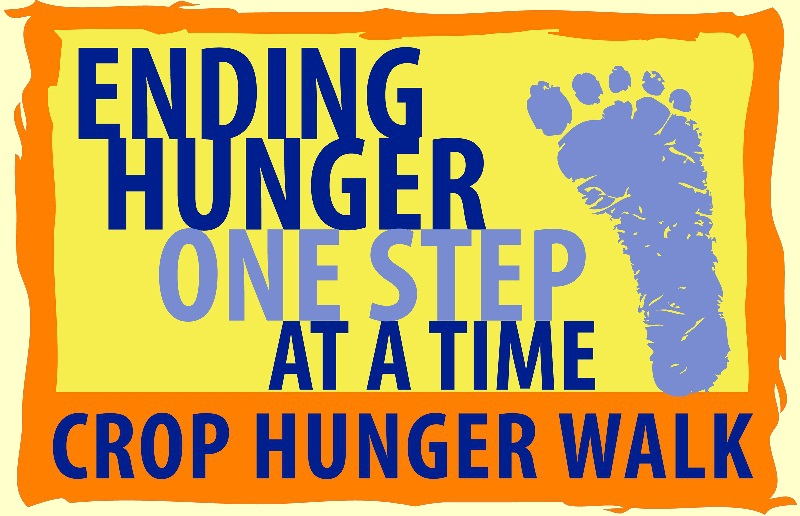Fight Bedtime Hunger
No child should go to bed hungry? You can help. Support local hunger agencies and the global hunger relief efforts of Church World Service. Join the Rockford CROP Hunger Walk at the Riverfront Museum on Sunday, October 20. Help us reach our goal of 200 walkers and \$25,000 raised! One o’clock Registration features music by Trinadora.

More information may be found at crophungerwalk.org/rockfordil. Every donation helps end hunger and save lives! Help us reach our goal of 200 walkers and \$25,000 raised! Walk in person or in spirit. Spirit walkers reserve your t-shirt by calling David Jones at 815-540-3703.

 table div table+table+table+table+table+table+table+table+table+table+table+table+table+table+table+table+table+table+table+table+table+table+table+table+table+table+table div table{width:100%;padding:0}table div table+table+table+table+table+table+table+table+table+table+table+table+table+table+table+table+table+table+table+table+table+table+table+table+table+table+table div table img{width:96.23%;padding:0;float:none}table div table+table+table+table+table+table+table+table+table+table+table+table+table+table+table+table+table+table+table+table+table+table+table+table+table+table+table div table td{width:100%;padding:0 1.88% 18px}/* styles */New Member Orientation
New Member Orientation will be Sunday, October 6, 13, 20 and 27 from 10:45-11:45 A.M. in the Cafe/ Conference room. If you have already made a decision to join St. Mark or would like to know more about St. Mark and the theology of the Evangelical Lutheran Church in America as you consider whether St. Mark is the church for you, we invite you to attend these coming sessions. The reception of new members is November 4 at the 9:30 A.M. service. Please contact Pastor Chad McKenna at 815-398-3557 or prmckenna@stmarklc.com if you would like to be included in the class list. Thank you.

 table div table+table+table+table+table+table+table+table+table+table+table+table+table+table+table+table+table+table+table+table+table+table+table+table+table+table+table+table+table div table{width:100%;padding:0}table div table+table+table+table+table+table+table+table+table+table+table+table+table+table+table+table+table+table+table+table+table+table+table+table+table+table+table+table+table div table img{width:96.23%;padding:0;float:none}table div table+table+table+table+table+table+table+table+table+table+table+table+table+table+table+table+table+table+table+table+table+table+table+table+table+table+table+table+table div table td{width:100%;padding:0 1.88% 18px}/* styles */Last Call for College CARE package addresses.
Each year we send CARE package to our St. Mark members that are at college or in the military. We know there are a lot of young people that would enjoy getting a CARE package. This is the last week to send in an address. Please e-mail Melinda@stmarklc.com

 table div table+table+table+table+table+table+table+table+table+table+table+table+table+table+table+table+table+table+table+table+table+table+table+table+table+table+table+table+table+table+table div table{width:100%;padding:0}table div table+table+table+table+table+table+table+table+table+table+table+table+table+table+table+table+table+table+table+table+table+table+table+table+table+table+table+table+table+table+table div table img{width:96.23%;padding:0;float:none}table div table+table+table+table+table+table+table+table+table+table+table+table+table+table+table+table+table+table+table+table+table+table+table+table+table+table+table+table+table+table+table div table td{width:100%;padding:0 1.88% 18px}/* styles */Day trip to Geneva, Illinois (near St. Charles) Invite your friends, neighbor, co-workers to join us November 16. We will travel by coach bus and the cost is \$35. Sign up in Fellowship Hall or call the church office 815-398-3557.

Geneva has beautiful shops, restaurants, art galleries, chocolate shops. Watch for more details as they become available.

 table div table+table+table+table+table+table+table+table+table+table+table+table+table+table+table+table+table+table+table+table+table+table+table+table+table+table+table+table+table+table+table+table+table div table{width:100%;padding:0}table div table+table+table+table+table+table+table+table+table+table+table+table+table+table+table+table+table+table+table+table+table+table+table+table+table+table+table+table+table+table+table+table+table div table img{width:96.23%;padding:0;float:none}table div table+table+table+table+table+table+table+table+table+table+table+table+table+table+table+table+table+table+table+table+table+table+table+table+table+table+table+table+table+table+table+table+table div table td{width:100%;padding:0 1.88% 18px}/* styles */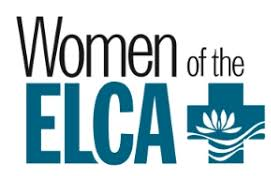Miriam Circle
Miriam Circle will meet on October 9 at 12:30 P.M. at Peterson Meadows. Wilma Reinke and Sue Larson are co-hosting.

Ruth Circle
Ruth Circle will meet at Stockholm Inn on Tuesday, October 10, at 9:30 AM. Maureen Hargrove will be our guest speaker as we learn more about St. Mark’s involvement with Habitat for Humanity. We will also tour a habitat home. All women of the church and invited guests are welcome. Please RSVP to Stella Schroeder: 815 282-5021 or caschroede@aol.com.

 table div table+table+table+table+table+table+table+table+table+table+table+table+table+table+table+table+table+table+table+table+table+table+table+table+table+table+table+table+table+table+table+table+table+table+table div table{width:100%;padding:0}table div table+table+table+table+table+table+table+table+table+table+table+table+table+table+table+table+table+table+table+table+table+table+table+table+table+table+table+table+table+table+table+table+table+table+table div table img{width:96.23%;padding:0;float:none}table div table+table+table+table+table+table+table+table+table+table+table+table+table+table+table+table+table+table+table+table+table+table+table+table+table+table+table+table+table+table+table+table+table+table+table div table td{width:100%;padding:0 1.88% 18px}/* styles */Property Notes
The top of the canopy is up on the north entrance. There is still some electrical work that needs to be completed.

We are aware of how dark the north side of the parking lot is in the evening. We have contacted the appropriate people and they are working on this.

 table div table+table+table+table+table+table+table+table+table+table+table+table+table+table+table+table+table+table+table+table+table+table+table+table+table+table+table+table+table+table+table+table+table+table+table+table+table div table{width:100%;padding:0}table div table+table+table+table+table+table+table+table+table+table+table+table+table+table+table+table+table+table+table+table+table+table+table+table+table+table+table+table+table+table+table+table+table+table+table+table+table div table img{width:96.23%;padding:0;float:none}table div table+table+table+table+table+table+table+table+table+table+table+table+table+table+table+table+table+table+table+table+table+table+table+table+table+table+table+table+table+table+table+table+table+table+table+table+table div table td{width:100%;padding:0 1.88% 18px}/* styles */Yoga is Happening at St. Mark!
Yoga can help build flexibility, strength, endurance, and coordination, as well as relieve stress and anxiety, and improve mindfulness. Using breath and movement, this flowing style of yoga is for both beginners and those with some yoga experience. Join us on Monday, October 7, at 4:30 pm in the Common Grounds Cafe. If you are interested, please sign-up in Fellowship Hall.

 table div table+table+table+table+table+table+table+table+table+table+table+table+table+table+table+table+table+table+table+table+table+table+table+table+table+table+table+table+table+table+table+table+table+table+table+table+table+table+table div table{width:100%;padding:0}table div table+table+table+table+table+table+table+table+table+table+table+table+table+table+table+table+table+table+table+table+table+table+table+table+table+table+table+table+table+table+table+table+table+table+table+table+table+table+table div table img{width:96.23%;padding:0;float:none}table div table+table+table+table+table+table+table+table+table+table+table+table+table+table+table+table+table+table+table+table+table+table+table+table+table+table+table+table+table+table+table+table+table+table+table+table+table+table+table div table td{width:100%;padding:0 1.88% 18px}/* styles */Altar Guild
The correct date for the next Altar Guild meeting is Wednesday, October 9 at 10:15 in the Common Grounds Cafe.

 table div table+table+table+table+table+table+table+table+table+table+table+table+table+table+table+table+table+table+table+table+table+table+table+table+table+table+table+table+table+table+table+table+table+table+table+table+table+table+table+table+table div table{width:100%;padding:0}table div table+table+table+table+table+table+table+table+table+table+table+table+table+table+table+table+table+table+table+table+table+table+table+table+table+table+table+table+table+table+table+table+table+table+table+table+table+table+table+table+table div table img{width:96.23%;padding:0;float:none}table div table+table+table+table+table+table+table+table+table+table+table+table+table+table+table+table+table+table+table+table+table+table+table+table+table+table+table+table+table+table+table+table+table+table+table+table+table+table+table+table+table div table td{width:100%;padding:0 1.88% 18px}/* styles */## Children's MinistrySunday School
We welcome all students to Sunday School, ages 3 through 6th grade. If you have not registered your student, please stop by the table in Fellowship Hall or you can register online. www.stmarklc.com/ministries. There is no fee for Sunday School, just an information sheet that we need for each child.

We are so excited to begin a new program this year, learning about God’s love and sharing it with the world!

 table div table+table+table+table+table+table+table+table+table+table+table+table+table+table+table+table+table+table+table+table+table+table+table+table+table+table+table+table+table+table+table+table+table+table+table+table+table+table+table+table+table+table+table+table div table{width:100%;padding:0}table div table+table+table+table+table+table+table+table+table+table+table+table+table+table+table+table+table+table+table+table+table+table+table+table+table+table+table+table+table+table+table+table+table+table+table+table+table+table+table+table+table+table+table+table div table img{width:96.23%;padding:0;float:none}table div table+table+table+table+table+table+table+table+table+table+table+table+table+table+table+table+table+table+table+table+table+table+table+table+table+table+table+table+table+table+table+table+table+table+table+table+table+table+table+table+table+table+table+table div table td{width:100%;padding:0 1.88% 18px}/* styles */## Youth Ministry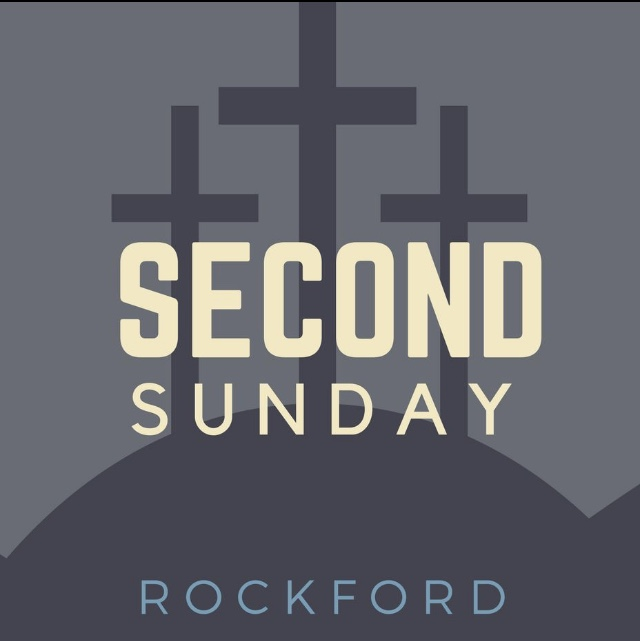Second Sunday is happening the second Sunday of each month. High School youth are leading worship, games, and music. Join us October 10th at 7:00 P.M. at Katie’s Cup. Need a ride? Have a question? Please message Melinda Alekna 815-871-0390.

 table div table+table+table+table+table+table+table+table+table+table+table+table+table+table+table+table+table+table+table+table+table+table+table+table+table+table+table+table+table+table+table+table+table+table+table+table+table+table+table+table+table+table+table+table+table+table+table div table{width:100%;padding:0}table div table+table+table+table+table+table+table+table+table+table+table+table+table+table+table+table+table+table+table+table+table+table+table+table+table+table+table+table+table+table+table+table+table+table+table+table+table+table+table+table+table+table+table+table+table+table+table div table img{width:96.23%;padding:0;float:none}table div table+table+table+table+table+table+table+table+table+table+table+table+table+table+table+table+table+table+table+table+table+table+table+table+table+table+table+table+table+table+table+table+table+table+table+table+table+table+table+table+table+table+table+table+table+table+table div table td{width:100%;padding:0 1.88% 18px}/* styles */## Barnabas Ministry

 table div table+table+table+table+table+table+table+table+table+table+table+table+table+table+table+table+table+table+table+table+table+table+table+table+table+table+table+table+table+table+table+table+table+table+table+table+table+table+table+table+table+table+table+table+table+table+table+table+table div table,table.module-48{width:3.77%;float:left;padding:0}table div table+table+table+table+table+table+table+table+table+table+table+table+table+table+table+table+table+table+table+table+table+table+table+table+table+table+table+table+table+table+table+table+table+table+table+table+table+table+table+table+table+table+table+table+table+table+table+table+table div table a{border:0 none;text-decoration:none}table div table+table+table+table+table+table+table+table+table+table+table+table+table+table+table+table+table+table+table+table+table+table+table+table+table+table+table+table+table+table+table+table+table+table+table+table+table+table+table+table+table+table+table+table+table+table+table+table+table div table img{width:100%!important;border:0 none;text-decoration:none}table div table+table+table+table+table+table+table+table+table+table+table+table+table+table+table+table+table+table+table+table+table+table+table+table+table+table+table+table+table+table+table+table+table+table+table+table+table+table+table+table+table+table+table+table+table+table+table+table+table div table td{width:100%;padding:0 20px 20px 0}/* styles */ Dear Friend – I was thinking…an elder named Bob said to me…about when I was a boy in the Depression. The only way we could have milk was to steal it. So when the milk truck came into the neighborhood we would jump in, steal some milk, and jump off. Know what I just realized? Every time the driver saw us he’d slow down for us. He let us do that. How many times was exactly the right person there for you? NOW, could you be that person for an elder at St. Mark? So many have no one and visiting just once a month could mean the world to them. You could be the ONLY visitor that month. Let me introduce you to an elder, enjoy, and meet – someone who needs you now. May the Lord watch…Pastor Chuck p.s. Please come to our next Reunion Group on Thursday, October 17 at 2:00 in the Conference room! And call me at 815-342-6064 for more info!
 table div table+table+table+table+table+table+table+table+table+table+table+table+table+table+table+table+table+table+table+table+table+table+table+table+table+table+table+table+table+table+table+table+table+table+table+table+table+table+table+table+table+table+table+table+table+table+table+table+table+table div table{width:100%;padding:0}table div table+table+table+table+table+table+table+table+table+table+table+table+table+table+table+table+table+table+table+table+table+table+table+table+table+table+table+table+table+table+table+table+table+table+table+table+table+table+table+table+table+table+table+table+table+table+table+table+table+table div table img{width:96.23%;padding:0;float:none}table div table+table+table+table+table+table+table+table+table+table+table+table+table+table+table+table+table+table+table+table+table+table+table+table+table+table+table+table+table+table+table+table+table+table+table+table+table+table+table+table+table+table+table+table+table+table+table+table+table+table div table td{width:100%;padding:0 1.88% 18px}/* styles */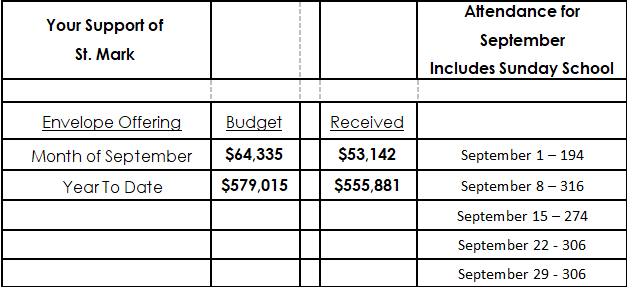table div table+table+table+table+table+table+table+table+table+table+table+table+table+table+table+table+table+table+table+table+table+table+table+table+table+table+table+table+table+table+table+table+table+table+table+table+table+table+table+table+table+table+table+table+table+table+table+table+table+table+table+table div table{width:100%;padding:0}table div table+table+table+table+table+table+table+table+table+table+table+table+table+table+table+table+table+table+table+table+table+table+table+table+table+table+table+table+table+table+table+table+table+table+table+table+table+table+table+table+table+table+table+table+table+table+table+table+table+table+table+table div table img{width:96.23%;padding:0;float:none}table div table+table+table+table+table+table+table+table+table+table+table+table+table+table+table+table+table+table+table+table+table+table+table+table+table+table+table+table+table+table+table+table+table+table+table+table+table+table+table+table+table+table+table+table+table+table+table+table+table+table+table+table div table td{width:100%;padding:0 1.88% 18px}/* styles */## Our St. Mark members:

Deb Lindley, Jim Tooley, Jerry Cazaniga, David Young, Keith Haggestad.

## Family and friends of St. Mark members:

Polly Young, Eva Love Sherbondy, Matthew and David Lundine, Betty Anderson, Jennifer Peterson, Lynn Reed, Dale Tallmadge, Pastor Jane McChesney, Earl Karau, Ann Eck, Clay Heinrich, Denise Nichols, Mary Graham, Barbara Heckart, Kim Menke, Norma Comstock, Ethan Whitney, Carolyn King, Tom Severson, Katie Butera, Ashton Henley, Nathan Gyllin.

## Those serving in the military and their families:

Tristan Davenport, Scot Hornick, Bailey Panjkovich, Xander Gehrke, Jim Tammen, Brandon Ballenger, Taylor Eversole, Dean Barron.

 table div table+table+table+table+table+table+table+table+table+table+table+table+table+table+table+table+table+table+table+table+table+table+table+table+table+table+table+table+table+table+table+table+table+table+table+table+table+table+table+table+table+table+table+table+table+table+table+table+table+table+table+table+table+table+table div table{width:100%;padding:0}table div table+table+table+table+table+table+table+table+table+table+table+table+table+table+table+table+table+table+table+table+table+table+table+table+table+table+table+table+table+table+table+table+table+table+table+table+table+table+table+table+table+table+table+table+table+table+table+table+table+table+table+table+table+table+table div table img{width:96.23%;padding:0;float:none}table div table+table+table+table+table+table+table+table+table+table+table+table+table+table+table+table+table+table+table+table+table+table+table+table+table+table+table+table+table+table+table+table+table+table+table+table+table+table+table+table+table+table+table+table+table+table+table+table+table+table+table+table+table+table+table div table td{width:100%;padding:0 1.88% 18px}/* styles *//* styles */ Office Hours Monday - Friday, 8:00 A.M. - 4:00 P.M. Telephone: 815-398-3557 Publications St. Mark Memos, the weekly e-newsletter, is distributed each Thursday. The deadline for submission is Monday by 4:00 P.M. Please click to send submissions. Sermons Online Miss a Sunday at St. Mark? Please visit our website and watch the sermons online. Please click to watch.